# Forced vibrations with damping

In the introductory tutorial in this series we considered a harmonic forcing function F0cos(ωt) applied to a translational spring and mass system with one degree of freedom having mass m, spring constant k  and a dashpot providing viscous damping with damping constant c shown in Figure 1 below with the spring extended by distance x .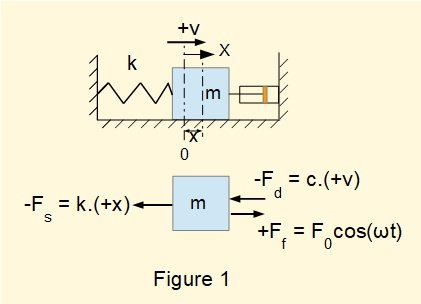From the dynamic* free body diagram we obtained the following equation of motion in terms of displacement x and time t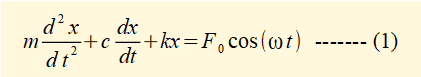*  showing forces producing acceleration of mass m, not static equilibrium

In the previous tutorial we found the particular solution* to the differential equation of motion for forced vibrations with a harmonic forcing function F0cos(ωt) without damping to be:where ωn is the natural frequency of the system and x0 and v0 are the initial conditions for displacement and velocity respectively.

* the maths tutorial in this series provides an outline of solutions to homogeneous and non-homogeneous second-order ordinary differential equations

The cos(ωnt) and sin(ωnt) terms in equation (2) express the free vibration and the cos(ωt) term expresses the steady state motion attributable to the harmonic forcing function.  Because of damping the transient cos(ωnt) and sin(ωnt) terms become zero leaving the cos(ωt) term expressing the steady state vibration.

In this tutorial we are interested in the steady state vibration as this has much greater practical significance.  Hence we only consider the particular solution for the non-homogeneous equation of motion (1).

In a previous tutorial we showed that the general solution to the equation of motion for free vibrations with damping is a function of cos(ωdt - φ) where ωd is the damped natural frequency and  φ the phase angle.   Thus a reasonable "guess" for the particular solution to equation (1) for steady state conditions is:

xp =  X.cos(ωt - φ) ----- (3)

where X is the amplitude of the steady state response and ω the angular frequency of the harmonic forcing function.  There are two unknowns in this equation, X and  φ to be resolved.

Now substitute xp, xp/ and xp// into equation (1)

xp =  X.cos(ωt - φ)        xp/  = -X.ω.sin(ωt - φ)          xp// = -X.ω2.cos(ωt - φ)    gives: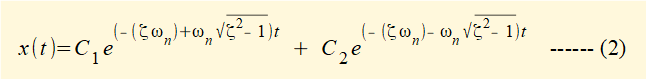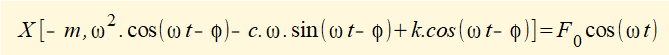dividing by k gives: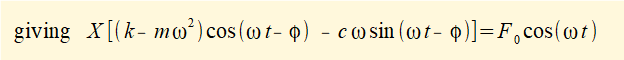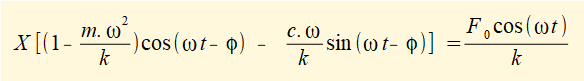* see a previous tutorialWe use the following trig identities to create two equations from (3).

(cos(ωt - φ) = cos(ωt).cos(φ) + sin(ωt).sin(φ)   and     sin(ωt - φ) = sin(ωt).cos(φ) - cos(ωt).sin(φ)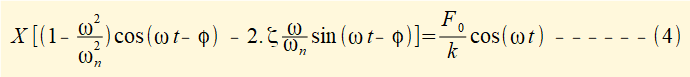X.cos(ωt).[A.cos(φ) + B.sin(φ)] + X.sin(ωt).[A.sin(φ) - B.cos(φ)] = (F0/k).cos(ωt)

which by equivalence of coefficients for cos(ωt) and sin(ωt) terms gives two equations:

X.cos(ωt).[A.cos(φ) + B.sin(φ)] =  (F0/k).cos(ωt)     and    X.sin(ωt).[A.sin(φ) - B.cos(φ)] = 0

which reduce to the following equations with unknowns X and φ

X.[A.cos(φ) + B.sin(φ)] = (F0/k) ---- (i)     X.[A.sin(φ) - B.cos(φ)] = 0 ---- (ii)

Summary solution:

multiply (i) by cos(φ) and (ii) by sin(φ) then add the resulting equations to get X.A = (F0/k).cos(φ) ---- (iii)

multiply (i) by sin(φ) and (ii) by cos(φ) then subtract resulting (i) - (ii) to get X.B = (F0/k).sin(φ) ---- (iv)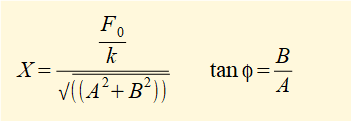square both sides of (iii) and (iv) and add the equations to give   X2 = (F0/k)2 / (A2 + B2)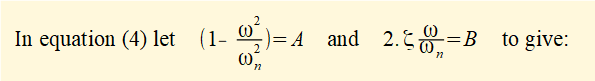substituting back for A and B gives: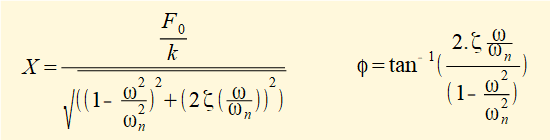Thus the steady state motion of a damped forced vibration with forcing function F0cos(ωt) is given by equation

x(t) =  X.cos(ωt - φ)   where X and φ are the above constants.

We now examine the characteristics of the motion in terms of  amplitude X, frequency ratio (ω/ωn) and phase angle φ.

In a previous tutorial we defined the static amplitude δ = (F0 / k) expressing the static displacement of mass m under force F0. Hence the amplification factor (X / δ) is a measure of the amplification effected by the forcing function for the steady state response x(t).

Figures 2 and 3 below show the response of the system for two particular sets of parameter values.Figure 2 shows displacement x(t) for a system where the frequency ω of the forcing function F0cos(ωt) is less than ωn the natural frequency  of the spring and mass system.   In this example the damping ratio ζ = 0.1 and ω/ωn = 2/3.  The amplification factor is greater than 1. x(t) lags the forcing function by phase angle φ.Figure 3 shows displacement x(t) for a system where the frequency ω of the forcing function F0cos(ωt) is greater than the natural frequency ωn of the system.   In this example the damping ratio ζ = 0.1 and ω/ωn = 4/3.  The amplification factor is greater than 1.  x(t) leads the forcing function by phase angle φ.

Figures 4 and 5 below illustrate a broader picture of the output characteristics.In Figure 4 the amplification factor is plotted against the frequency ratio for three values of damping ratio ζ.  The amplification ratio peaks at frequency ratio = 1 which is also the resonant condition for the undamped system derived in the previous tutorial.  The lower the value of ζ the higher the amplification factor at all frequency ratios.  Note that at frequency ratios < 1 the amplification factor is always  > 1.  As frequency ratios increase beyond the peak value the amplification factor reduces progressively to become < 1 and ultimately tends to zero.  These characteristics are similar to the undamped system.

Note the marked points for frequency ratios used in Figures 2 and 3 for ζ = 0.1.Figure 5 shows the variation of phase angle (units in radians) with frequency ratio for three values of damping ratio ζ.  The principal characteristics are:

• At frequency ratios < 1 displacement x(t) lags the forcing function and the phase angle increases as the frequency ratio increases.
• When the frequency ratio = 1 the phase angle is 90°.
• At frequency ratios >1 displacement x(t) leads the forcing function and the phase angle increases as the frequency ratio increases, tending to 180° .  This characteristic is more pronounced the lower the damping ratio.

Note the marked points for frequency ratios used in Figures 2 and 3 for ζ = 0.1.

The phase angle "flips" when ratio ω/ωn  transitions through the value 1.  Figures 6 and 7 below show phase angles respectively at frequency ratios just less than 1 and just greater than 1.This tutorial concludes the series on mechanical vibrations for the present.  I plan to continue the series to cover torsional vibrations, multiple degree of freedom systems and transverse vibration of beams.

I welcome feedback at:

### Tutorials - mechanical vibrations

###### Forced vibrations with damping

Alistair's tutorials 2021